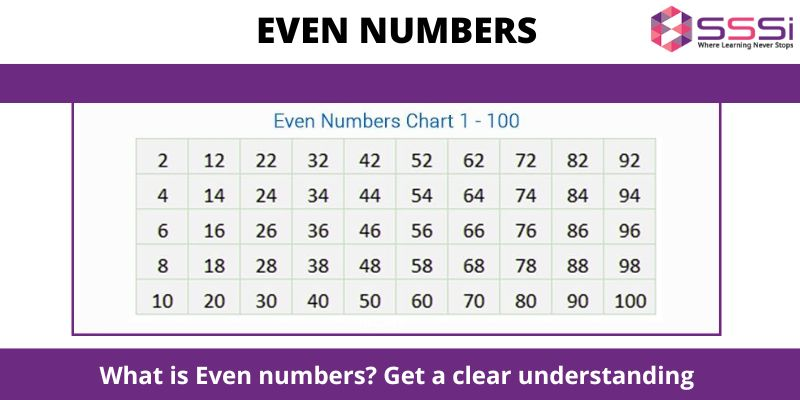# What is Even numbers? Get a clear understandingAn even number is any integer that can be divided by 2 precisely. The final digit of an even integer is always one of the following: 0, 2, 4, 6, or 8. Even numbers can include 2, 4, 6, 8, 10, 12, and 14. These are even numbers because they are simple to divide by two. Precisely, keep in mind that 2 is the minimum positive even natural number. An odd number is one that you choose that cannot be split by two. Examples are 1, 3, 5, 7, 9, etc.

Thus, even and odd numbers may be used to categorise all numbers. It follows that a number must either be even or odd. Online maths tuition class ptoviders can help you to understand the main concept of these numbers.

## Understand the process to determine a odd or even number

You must examine the supplied number in its position of one (or unit) to see whether it is odd or even. You may determine if a number is odd or even by substituting that specific number in its place.

The last even number is 0, 2, 4, 6, or 8.

Odd The numbers are 1, 3, 5, 7, and 9.

These are some easy methods for determining if a number is even or not.

Consider the number 3, 845, 917, which has an odd number at the conclusion (i.e., 3, 5 and 7). The digits 3, 845, and 917 are odd numbers as a result. As a result, the number is not even. Similar to how 8 is an even number since it finishes in 8 and 2, 322 is also. A number is said to be even if it can be divided into two groups with an equal number of components in each group. When grouping odd numbers, we obtain a residual of 1. A number is considered even if it repeatedly divides into groups of "two" without leaving any residue. A number is an odd number if there is a remainder.

## What are the even numbers from  1 to 100?

Class 9 maths students get these types of numbers while doing calculations and sums. It is a human tendency to forget thing. So, here we have added the even numbers between 1 to 100. Take a look at it  - 2, 4, 6, 8, 10, 12, 14, 16, 18, 20, 22, 24, 26, 28, 30, 32, 34, 36, 38, 40, 42, 44, 46, 48, 50, 52, 54, 56, 58, 60, 62, 64, 66, 68, 70, 72, 74, 76, 78, 80, 82, 84, 86, 88, 90, 92, 94, 96, 98, 100.

## Properties of even numbers

If you add even and ood numbers then the result would always be odd.

Ex: 5 + 5 = 10,

5 + 18 = 23

If you add even and even number  then the result will also be even.

Ex: 14+10 = 24

If you add odd and odd then the result will always be even.

Ex: 13 + 7 = 20

Property of Subtraction

Subtract even from odd (or vice-versa), the resulting number is always odd.

Ex: 7 – 4 = 3,

10 – 5 = 5

Subtract even from even, the resulting number is always even.

Ex: 14 – 4 = 10

Subtract odd from odd, the resulting number is always even.

Ex: 23 – 13 = 10

Property of Multiplication

Multiply even and even will always result in an even number.

Ex: 6 × 4 = 24,

12 × 4 = 48

Multiply even and odd numbers will result in an even number.

Ex: 4 × 5 = 20,

6 × 3 = 18

Multiply odd and odd numbers will give an odd number always.

Ex: 3 × 5 = 15,

5 × 9 = 45

In short we can calculate the properties of even numbers are given below -

• Two even integers added together equal an even number.
• Two odd integers added together equal an even number.
• Even and an odd number added together equal an odd number.
• When an even integer is divided by 2, there is no residue.
• Because an odd number cannot be divided by two in its whole, one remains.
• When a number is even, it finishes in 0, 2, 4, 6, and 8.
• Odd numbers have the last digits 1, 3, 5, 7, and 9.

## What are Even and Odd Decimals?

Due to the fact that decimals are not whole numbers, they are neither even nor odd. For instance, you cannot classify a fraction as odd or even just on the denominator, as in the case of the fractions 1/3 and 12.34, respectively. Decimals and fractions cannot be even or odd since only integers may be even or odd. However, since zero is an integer and a multiple of two, it is even. Odd numbers are ones that can't be divided by 2 evenly.

You know, taking  Online Coaching for maths can help you to deal with all your sums. Fell free to connect with our team for online tutoring services.